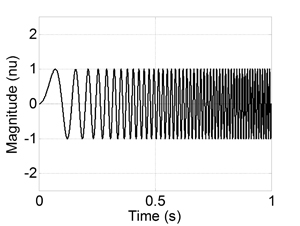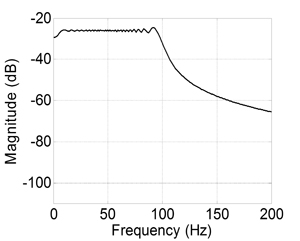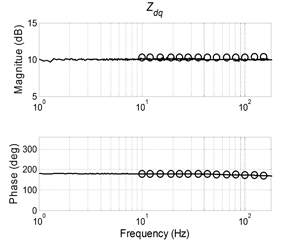LIBRARY

# Three-phase AC System Impedance Measurement Unit (IMU) using Chirp Signal Injection

Year: 2013Fig. 1. (a) Chirp signal example.
Power electronics technology has infiltrated the power processing and generation fields in contemporary electric power systems. Power electronic technology provides exceptional performance the negative incremental impedance characteristics of the converters introduces the possibility of system instability. Similar to DC power systems, the stability in balanced three-phase AC systems can be analyzed using the output and input impedances at the interface in the synchronous d-q coordinates. Within this scenario, there is a need to fully characterize the impedances of the various AC interfaces in electrical power systems.

The frequency sweep method widely used in literature takes a long time to extract the whole impedance curve, especially at low frequency range. This paper proposes using a chirp signal, which is one kind of wide bandwidth signal, to increase the measurement speed.

Figure 1 shows an example of chirp signal. Compared to other wide bandwidth signals, it has a low crest factor and a controlled spectrum shape.

As a wide bandwidth signal, the chirp signal spreads the energy over a frequency range, which results in a lower SNR compared to the sinusoidal signal used in a frequency sweep. A corresponding data procedure used with the chirp signal is developed. It includes oversampling the raw signals to increase the analog-to-digital conversion resolution, cross correlation to remove the non-correlated noise, and frequency domain averaging to further improve the signal SNR. The method is tested with both linear and nonlinear load. Figure 2 shows the measurement result of a diode rectifier when compared to the simulation result, which is extracted using a frequency sweep method. The two results show a good match.Fig. 1. (b)Fig. 2. (a) Input impedance of a diode rectifier
(Solid line: measurement result; circles: simulation result).Fig. 2. (b)Figure 2. (c)Figure 2. (d)